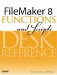# Sum()

Category: Aggregate

 Syntax: Sum ( field {; field...} )

Parameters:

fieldAny related field, repeating field, or set of non-repeating fields that represent a collection of numbers. Parameters in curly braces { } are optional.

Data type returned: Number

Description:

Returns the sum of all valid values represented by the fields in the parameter list.

The Sum() function is most often used to add up a column of numbers in a related table.

Examples:

Function

Results

Sum (field1; field2; field3)

Returns 6 (when field1 = 1, field2 = 2, and field3 = 3).

Sum (repeatingField)

Returns 6 (when repetition 1 = 1, repetition 2 = 2, and repetition 3 = 3).

Sum (repeatingField1; repeatingField2)

Returns a repeating calculation field where the first value equals the sum of the values in repeatingField1, and the second repitition contains the sum of the values in repeatingField2.

Sum (Customer::InvoiceTotal)

Returns 420 (when the sum of InvoiceTotal in the related set of data is 420).FileMaker 8 Functions and Scripts Desk Reference
ISBN: 0789735113
EAN: 2147483647
Year: 2004
Pages: 352